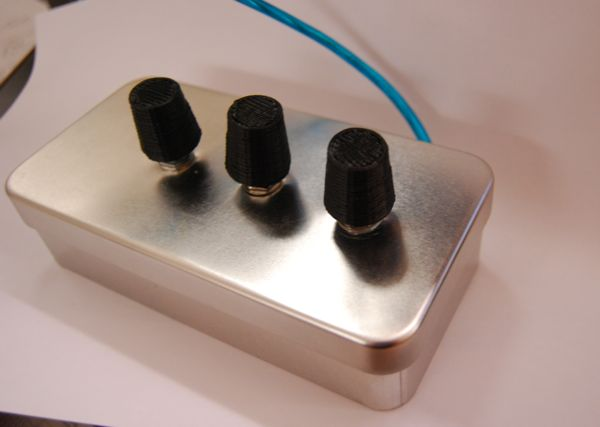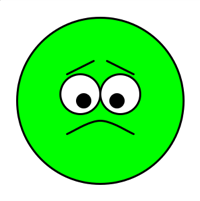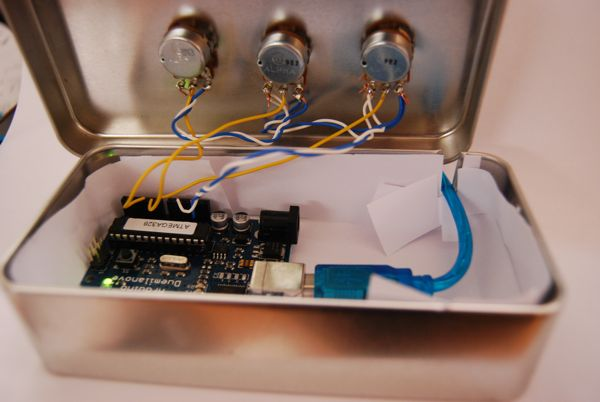## Smiley-O-Mat

I just finished my Smiley-O-Mat - it's a tin-box with 3 turning knobs that allows you to controll the appearence of a smiley. With 3 simple turns I can now tell your co-workers, familymembers, ... how you feel at the moment and if it is wise to talk to me right now :-)

The controllerbox is made with an arduino, the smiley generator is written in processing, and the turning knobs are printed on my makerbot

This is what the controller looks likeand here are some smileys i generatedThe box contains an arduino that is wired to three variable resistors with 100kOhm the middle connectors (yellow wire) go to analog 0, 1 and 2 the white one is ground and the blue one is +5Vthis is the code that runs on the arduino

``````void setup() {
Serial.begin(9600);
}

void loop() {
if (Serial.available() > 0) {
if ( in == 'a' ) {
Serial.print( "a " );
Serial.println((a1 + a2 + a3)/3);

} else if ( in == 'b' ) {
Serial.print( "b " );
Serial.println((b1 + b2 + b3)/3);

} else if ( in == 'c' ) {
Serial.print( "c " );
Serial.println((c1 + c2 + c3 )/3);

}
}
}
``````

and this is the processing code that draws the smiley

``````import processing.serial.*;

Serial ser;
int mouth = 0;
int eye = 0;
color c = color(255, 255, 0 );

void setup() {
ser = new Serial(this, Serial.list(), 9600);
size(300,300);
smooth();
background(255);

}

void draw() {

if ( val > -1 ) {
if (val < 512) {
c = color( map(val, 0, 512, 0, 255), 255, 0 );
} else {
c = color( 255, map(val, 512, 1024, 255, 0), 0 );
}
}
background(255);

fill(c);
ellipse(150,150,250,250);
strokeWeight(3);

int tmp = readPort( 'c' );
if ( tmp > -1 ) {
mouth = tmp;
}
if ( tmp > -1 ) {
eye = tmp;
}

fill(255);
ellipse( 120, 140, 60, 60);
ellipse( 180, 140, 60, 60);
fill(0);
ellipse( 125, 150, 20, 20);
ellipse( 175, 150, 20, 20);

line( 100, 100 + map( eye, 0, 1024, -10, 10), 140, 100 - map( eye, 0, 1024, -10, 10) );
line( 160, 100 - map( eye, 0, 1024, -10, 10), 200, 100 + map( eye, 0, 1024, -10, 10) );

noFill();
beginShape();
curveVertex(100, 200);
curveVertex(100, 200);
curveVertex(150, 180 + map( mouth, 0, 1024, 0, 50));
curveVertex(200, 200);
curveVertex(200, 200);
endShape();

}

int readPort( char port ) {
ser.write(port);
for( int i = 0; i < 50; i++ ) {
delay(10);
if (ser.available() > 0) {
if (in != null) {
try {
String intrim = in.trim();
if (intrim.charAt(0) == port ) {
int val = Integer.parseInt(intrim.substring(intrim.indexOf(" ")+1));
return val;
}
} catch (Exception e) {}
return -1;
}
}
}
return -1;
}
``````